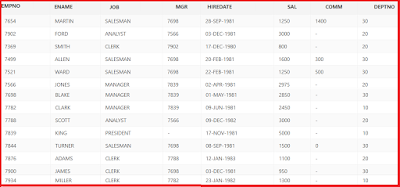# Index in SQL with examples

## Data Storage:

1. The data in SQL Server is stored in the form of data pages.
2. The size of each data page is 8kb.
3. The data page contains the page header which is 96 bytes.
4. The header will have previous pointer (PP), object id, next pointer (NP).
5. The previous pointer pointing to the previous page, this value is NULL for first page.
6. The next pointer pointing to the next page, it’s value is NULL for the last page.

## Data Access:

In SQL Server Data will be accessed in two ways.

Table Scan : It starts from beginning of the table and scan each and every page for required data, it extracts the rows to meet the query criteria.

Using Index: When index is created for a table, it travels through index tree structure to determine the required rows and extracts the rows which will meets the query criteria.

# User Defined Functions in SQL Server

The functions created by the user are called user defined functions.

Types of user defined functions:

1. Scalar Valued Functions
2. Table Valued Functions

## 1. Scalar Valued Functions:

The functions which return scalar (single value) to the calling environment are called scalar valued functions.

Syntax:
```create function (function_name)
(@para1 datatype(size), ……@para n datatype (size))
retrun datatype
as
begin
function body
return ( variable)
end
```
For executing the function
```Declare @ var (datatype)
Set @var= owner.funciton_name ( para1, para2..)
print@var
```
Example: Write a function to find the sum of the two numbers
```Create function add_sum ( @a int, @b int)
Return int
As
Begin
Declare @c int,
Set @c = @a+@b;
return(@c);
end
```
Executing the function
```Declare @z int
print@z
```

# Aggregate and Group Functions in SQL and Oracle with examples

• Aggregate functions will acts group of values at a time and returns a single value. It is also called Group functions.
• Aggregate functions will ignore NULL values while calculating.
• Here is the sample emp table using for solving examples.Emp Sample Table
1. Sum:
Sum is used to find the sum of values in a column.

Example:Write a query for find the total salary of emp table.
`select sum(sal) from emp`
2. Avg: Avg is used to find average values for given values.

Example:Write a query for find the average salary of emp table.

`select avg(sal) from emp`